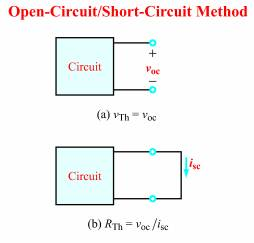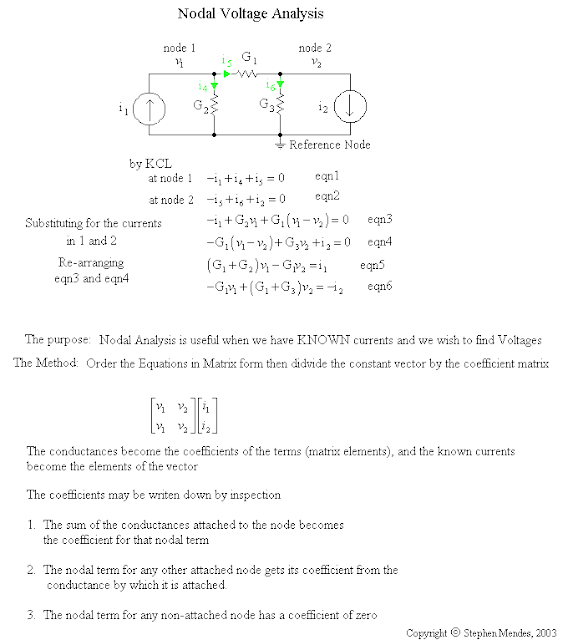## Thursday, June 23, 2011

### Network Analysis for Electric Circuits

Network Analysis for electric circuits are the different useful techniques related to several currents, emfs, and resistance voltages in such circuit. This is somewhat the collection of techniques of finding the voltages and currents in every component of the network. Some of those techniques are already mentioned in this online tutorial of Electrical Engineering.

There are six remaining useful techniques that we are going to learn. The practical example of each analysis will be given in my next post. This is for you to comprehend first what each theory is all about. So, let's begin the first useful technique in analyzing network.

Thevenin's Theorem

Consider the figure below which schematically represents the two-terminal network of constant emf's and resistances; a high-resistance voltmeter, connected to the accessible terminals, will indicate the so called open circuit voltage voc. If an extremely low-resistance ammeter is next connected to the same terminals, as in fig.(b), which is so called the short-circuit current isc will be measured.
﻿﻿﻿﻿﻿Test circuits for Thevenin's Theorem
Now the two quantities determined above may be used to represent an equivalent simple network consisting of the single resistance RTH, which is equal to voc/isc. If the resistor RL is connected to the two terminals, the load current of the circuit will be

IL = voc / RTH+RL---------------> equation no.1

The analysis leading to the equation no.1 above was first proposed by M.L. Thevenin the latter part of the nineteenth century, and has been recognized as an important principle in electric circuit theory. His theory was stated as follows: In any two-terminal network of fixed resistances and constant sources of emf, the current in the load resistor connected to the output terminals is equal to the current that would exist in the same resistor if it were connected in series with (a) a simple emf whose voltage is measured at the open-circuited network terminals and (b) a simple resistance whose magnitude is that of the network looking back from the two terminals into the network with all sources of emf replaced by their internal resistances.

Thevenin's Theorem has been applied to many network solutions which considerably simplify the calculations as well as reduce the number of computations.

Norton's Theorem

From the previous topic above, it was learned that a somewhat modified approach of Thevenin was formulated. This modified approach is to convert the original network into a simple circuit in which a parallel combination of constant-current source and looking-back resistance "feeds" the load resistor. Take a look on the figure below

﻿Norton's equivalent circuit
Take note that Norton's theory also make use of the resistance looking back into the network from the load resistance terminals, with all potential sources replaced by the zero-resistance conductors. It also employs a fictitious source which delivers a constant current, which is equal to the current that would pass into a short circuit connected across the output terminals of the original circuit.

From the fig (b) above of Norton's equivalent circuit, the load current would be

IL = IN RN / RN+RL ---------------> equation no.2

Superposition Theorem

The theorem states like this: In the network of resistors that is energized by two or more sources of emf, (a) the current in any resistor or (b) the voltage across any resistor is equal to: (a) the algebraic sum of the separate currents in the resistor or (b) the voltages across the resistor, assuming that each source of emf, acting independently of the others, is applied separately in turn while the others are replaced by their respective internal values of resistance.

This theorem is illustrated in the given circuit below:Illustration of Superposition Theorem
The original circuit above ( left part ) have one emf source and a current source. If you like to obtain the current I which is equal to the sum of I' + I"using the superposition theorem, we need to do the following steps:

a. Replace the current source Io by an open circuit. Therefore, an emf source vo will act independently having a current I' as the first value obtained when the circuit computed.

b. Replace emf source vo by a short circuit. This time Io will act independently and I" now will be obtained when the circuit computed.

c. The two values obtained ( I' and I") with emf and current source acting independently will be added to get I = I' + I"

Maxwell's Loop (Mesh) Analysis

The method involves the set of independent loop currents assigned to as many meshes as exists in the circuit, and these currents are employed in connection with appropriate resistances when Kirchhoff voltage law equations are written. Take a look on the given circuit below.Given circuit can be analyze using mesh method
The given circuit above have two voltage sources V1 and V2 are connected with a five-resistor network in which there are two loop currents i1 and i2. Observe that they are shown directed clockwise, a convention that is generally adopted for convenience. The following Kirchhoff's voltage-law may now be written as:

-V1 + i1R1 + R3(i1-i2) + i1R2 = 0     (loop 1)
-V2 + i2R5 +R3(i2-i1) + i2R4 = 0     (loop 2)

You may simplify the equations by using the simple algebra. This will be well explained on my next post for more practical examples of Network Analysis.

Nodal Analysis

For this analysis, every junction in the network that represents a connection of three or more branches is regarded as a node. Considering one of the nodes as a reference or zero-potential point, current equations are then written for the remaining junctions, thus a solution is possible with n-1 equations, where n is the number of nodes.

There are three basics steps to follow when using nodal analysis

a. Label the node voltages with respect to ground.
b. Apply KCL to each of the nodes in terms of the node voltages.
c. Determine the unknown node voltages by solving simultaneous equations from step b.

Take a look on the snapshot on how nodal analysis is being done. This is illustration by Stephen Mendez.  Don't worry I will give you the technique on my next post on how to solve nodal analysis.

Note: In the snapshot below, he used conductance which is G = 1/R.

﻿The Nodal Analysis Snapshot

Millman's Theorem

Any combination of parallel-connected voltage sources can be represented as a single equivalent source using Thevenin's and Norton theorems appropriately. This can be illustrated as :This is Millman's Theorem
The formula above can be written as:

VL = V1/R1 + V2/R2 + .....Vn / Rn
-------------------------------
1/R1 + 1/R2 + ......1/Rn +  1/RL

where:

V1, V2, V3... Vn  are the voltages of the individual voltage sources.
R1, R2, R3... Rn  are the internal resistances of the individual voltage sources.

I think this is enough for today. Don't forget to read my next post for more  practical examples regarding Network Analysis/Theorems.

Cheers!

haris said...

i am a student of electrical engineering and this information is really helpful for me , thanks.

Anonymous said...

yes it is too good enough

Rovanni Tagactac said...

pls give solved problems using these different techniques... tnx

brutumbo said...

this is very useful blog...
i wish you can frequently update this blog...
BTW pls post sample problems(with solution) using these different techniques...

ran daniels said...

Electric can get really complicated you really know your stuff

Anonymous said...

When are you going to do yout next Blog update

NORM

Anonymous said...

Hi I am a student taking calculus 1 and I got a strange idea that I could better understand the amount of amps needed to run a marine radio and speakers if I looked at them like a combination of functions. This hit me as I was reading about limit laws. After some google work, I found my self asking what is network analysis and is that of any help to me. After seeing the matrix in the examples I am wondering if I shouldnt wait until linear algebra. Thanks

amin said...

The content of your site seems very helpful for me.I am engineering student.Hope this will help me a lot.Thanks

The Mighty said...

This is a good article, some useful information. Good for beginning engineers.

Hello Engineers said...

Hey i am student of electronics engineering and i found this information quite helpful. Thank you :)

Unknown said...